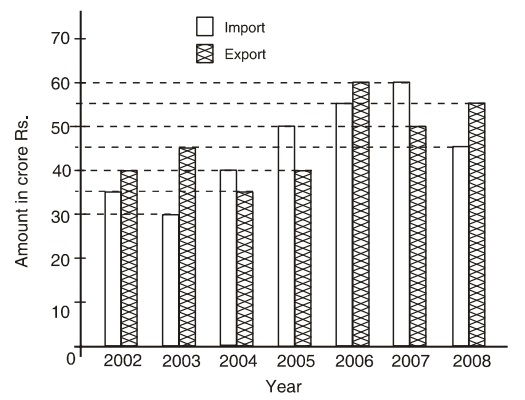## Bar graph

#### Data Interpretation

Direction: Study the following graph carefully to answer the questions given below it.
Imports and Exports of spare parts by an automobile company over the given years.1. During which year the percentage rise/fall in imports from the previous year is the lowest?

1. As per given graph , calculate the difference between two consecutive year change in import.

##### Correct Option: D

As per given graph, we calculate the difference in two consecutive years import.
In 2003, change in imports = (35 - 30)/35 x 100 = 100/7 = 139/7% fall
In In 2004, change in imports = (40 - 30)/ 50 x 100 = 1/3 x 100 = 331/3% rise
In 2006, change in imports = (55 - 50)/50 x 100 = 10% rise
In 2007, change in imports = (60 - 55/55) x 100 = 91/11% rise
Hence, in 2007 the percentage rise/fall in imports from the previous year is the lowest.

1. In which of the following pair of year and the percent increase in the export over the previous year is correctly matched?

1. As per given graph, calculate the total export one by one year which is given in answer and compare the result.

##### Correct Option: D

In 2002-03, Total import = 35 + 30 = Rs. 65 crores
Total export = 40 + 55 = Rs. 95 crores
In 2004-05 . Total import = 40 + 50 = Rs. 90 crores
Total export = 35 + 40 = Rs. 75 crores
In 2005-06, Total import = 40 + 50 = Rs. 90 crores
Total export = 40 + 60 = Rs. 100 crores
In 2007-08, Total import = 60 + 45 = Rs. 105 crores
Total export = 50 + 55 = Rs. 105 crores
Hence, in 2007-08 pair of years the total import is equal to total export.

1. Which of the following pair of year and the percent increase in the export over the previous year is correctly matched?

1. Percentage increase in export = (previous year export - current year export) x 100/current year export.
Use the above formula and compare their result for all given year in the question.

##### Correct Option: C

Percentage increase in export = (previous year export - current year export) x 100/current year export.
In 2003.
Percentage increase in export = (45 - 40) x 100 /40 = 12.5%
In 2004. there is decrease in export over the previous year.
In 2005.
Percentage increase in export = (40 - 35) x 100/35 = 14.29%
In 2008.
Percentage increase in export = (55 - 50) x 100/ 50 = 10%

The answer will be year 2005 and 14.2. The correct option is (c).

1. What is the ratio of total imports to the total exports for all the given years together ?

1. Total import = Add all the Import value from graph fro all the years.
Total export = Add all the Export value from graph fro all the years.

##### Correct Option: C

Total import = 35 + 30 + 40 + 50 + 55 + 60 + 45 = Rs. 315 crores
Total export = 40 + 55 + 35 + 40 + 60 + 50 + 55 = Rs. 335 crores
Since, their ratio = 315 : 335 = 63 : 65

1. The total export in the years 2004, 2005 and 2008 together are what percent of the total imports during the same period?

1. In 2004, 2005 and 2008, total import = Add imports value from graph for these years.
In 2004, 2005 and 2008, total export = Add export value from graph for these years.

##### Correct Option: C

In 2004, 2005 and 2008, total import = 40 + 50 + 45 = Rs. 135 crores
In 2004, 2005 and 2008, total export = 35 + 40 + 55 = Rs. 130 crores
Required percentage = 130/135 x 100 = 96.3%